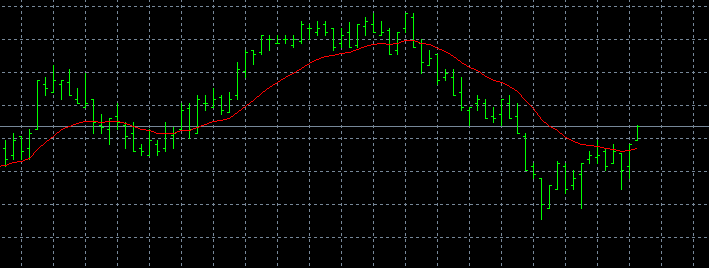# MA Exponential (iMA Method 1) and MA Smoothed (iMA Method 2) gives with some period combinations exact the same result.15

Hello dear programmers,

I made an expert advisor which uses 2 MA's (among some filters) to trade on the buy/sell signals when the MA-lines are crossing which looks like this:

double S0 = iMA(NULL, 0,MAC_MA_ShortPeriod,0,MAC_MA_ShortMode,PRICE_CLOSE,0);
double L0 = iMA(NULL, 0,MAC_MA_LongPeriod, 0,MAC_MA_LongMode, PRICE_CLOSE,0);
double S1 = iMA(NULL, 0,MAC_MA_ShortPeriod,0,MAC_MA_ShortMode,PRICE_CLOSE,1);
double L1 = iMA(NULL, 0,MAC_MA_LongPeriod, 0,MAC_MA_LongMode, PRICE_CLOSE,1);
PrintFormat("%s INFO: TickNo %d: S0=%.2f, L0=%.2f, S1=%.2f, L1=%.2f", __FUNCTION__, TickNo, S0, L0, S1, L1);

S0 is short Period current Bar, L0 is long Period current Bar, S1 is short Period previous Bar and L1 is long Period previous Bar.

MAC stands for MA-Crossing. The MA Periods and Modes are input variables.

The crossing is detected by this code:

if ((S0 > L0+INTG) && (S1 < L1-INTG)) NewSignal=+1;

if ((S0 < L0-INTG) && (S1 > L1+INTG)) NewSignal=-1;

I know the round brackets are not necessary here, but I'm custom to use that for clarity and safety.

INTG is my Indicator No Trade Gap variable to filter out some scatter.

With some short/long Period/Mode combinations like 9-2 17-1 or 17-1 9-2 (Period and Mode reversed) but not with 9-1 17-2 and 17-2 9-1 MT4 gives me the same result for the 2 MA's.

Here you see the result of my PrintFormat statement from the first piece of code I showed:

TickNo 1: S0=12200.38, L0=12200.38, S1=12201.57, L1=12201.57
TickNo 2: S0=12200.21, L0=12200.21, S1=12201.57, L1=12201.57
TickNo 3: S0=12200.04, L0=12200.04, S1=12201.57, L1=12201.57
TickNo 4: S0=12200.21, L0=12200.21, S1=12201.57, L1=12201.57
TickNo 5: S0=12200.38, L0=12200.38, S1=12201.57, L1=12201.57

You see that there is no difference between the short and the long period for Bar-0 and Bar-1. How about that? Twilight zone? Of course the result is one MA line and no trades.

This symptom occurs with every symbol, timeframe or date. The only dependency is the combination of periods with the modes 1 and 2.

Some other combitions I found are: 9-1 5-2, 11-1 6-2, 7-2 13-1, 6-2 11-1. But there are more.

Has anybody a clue how this can happen?